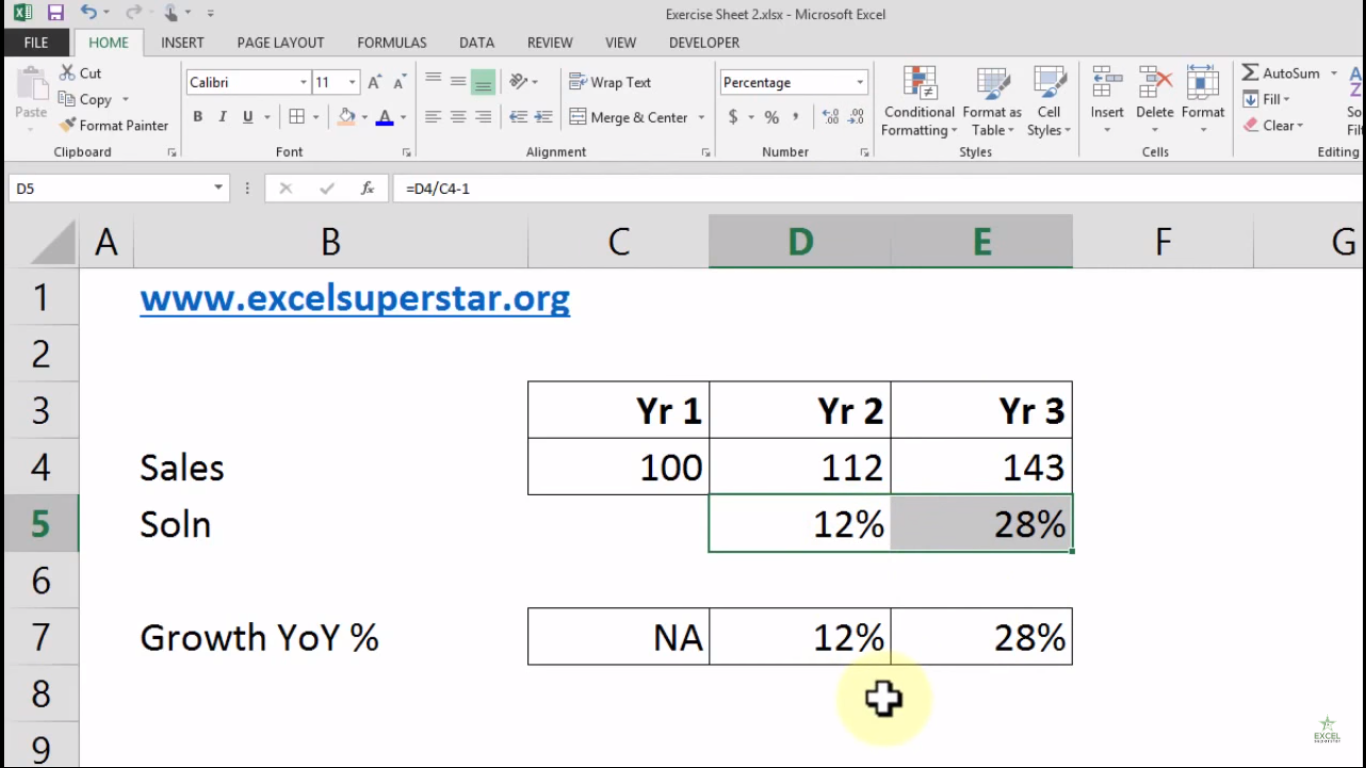# Excel Growth Calculating FunctionIn this tutorial, we will learn about Calculating Year on Year Growth % in Excel

Example:

There is a table consisting of 3 years of sales number and I want to calculate Year on Year Growth Percentage for all 3 years.

So to calculate the growth, follow the steps given below:

1. Write = and choose the current year sales number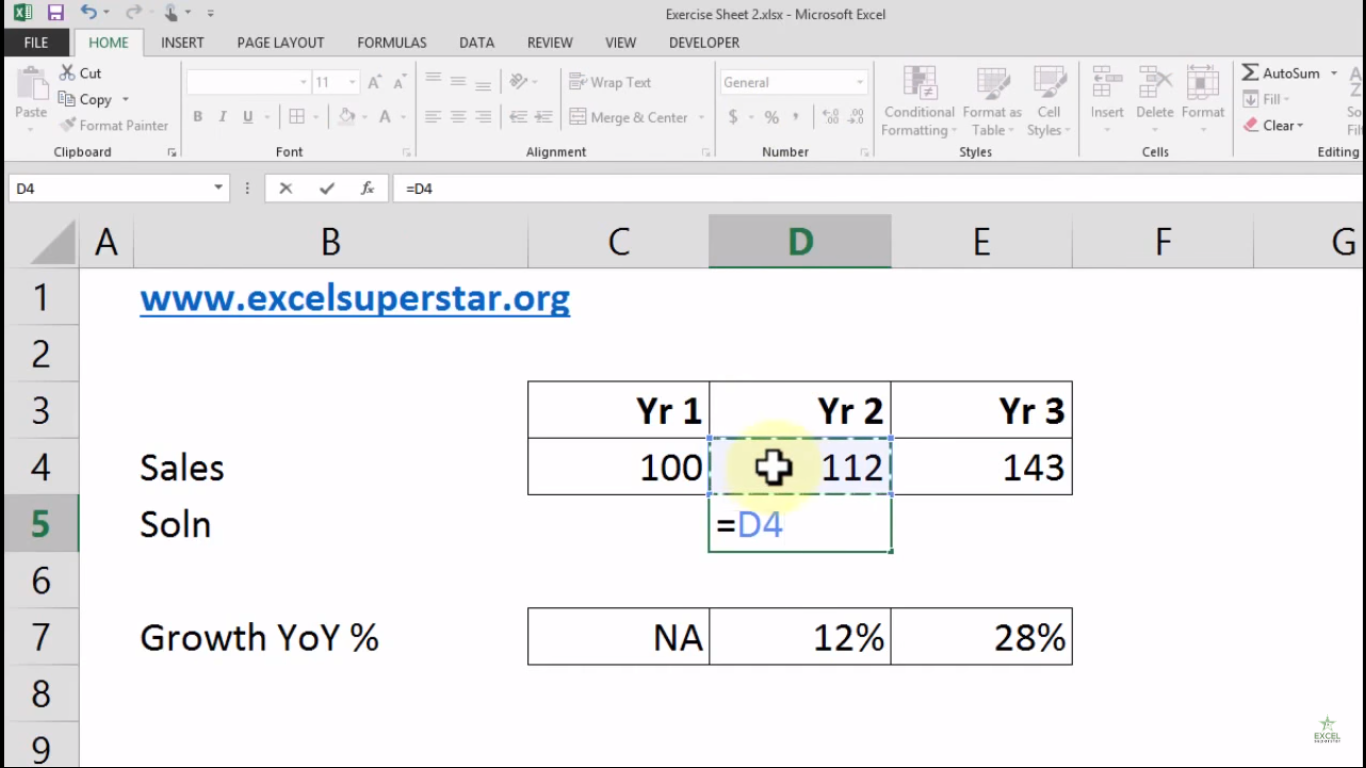2. Divide it by choosing the last year sales number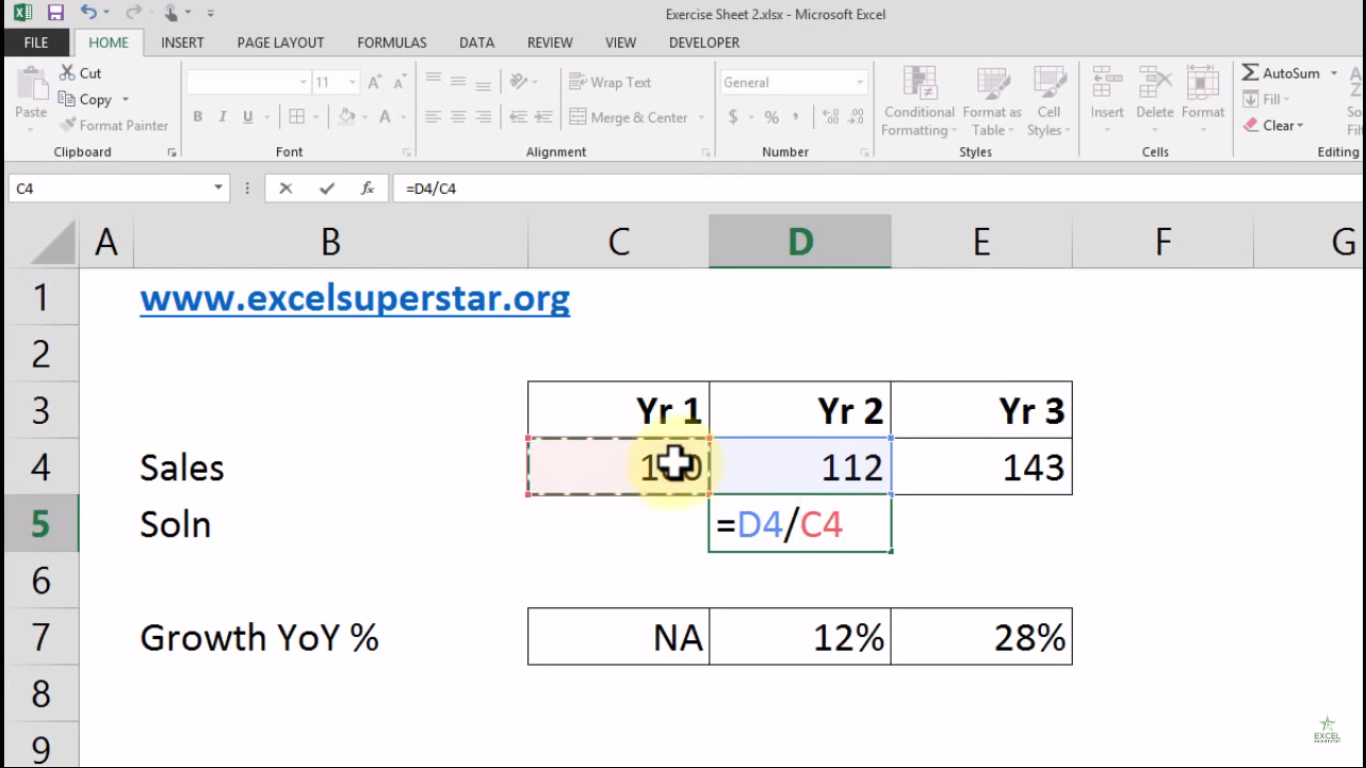3. At the end, write -1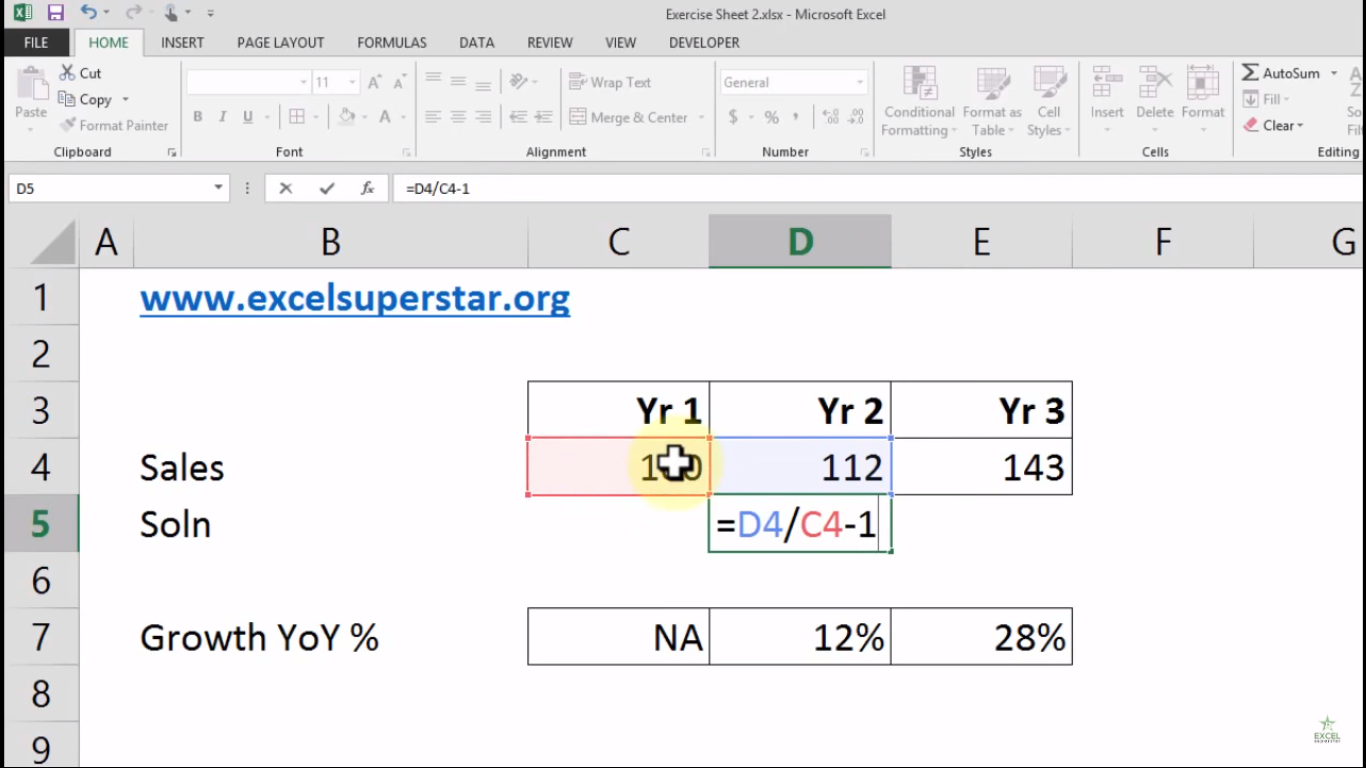4. Press Enter and the answer is 0.12%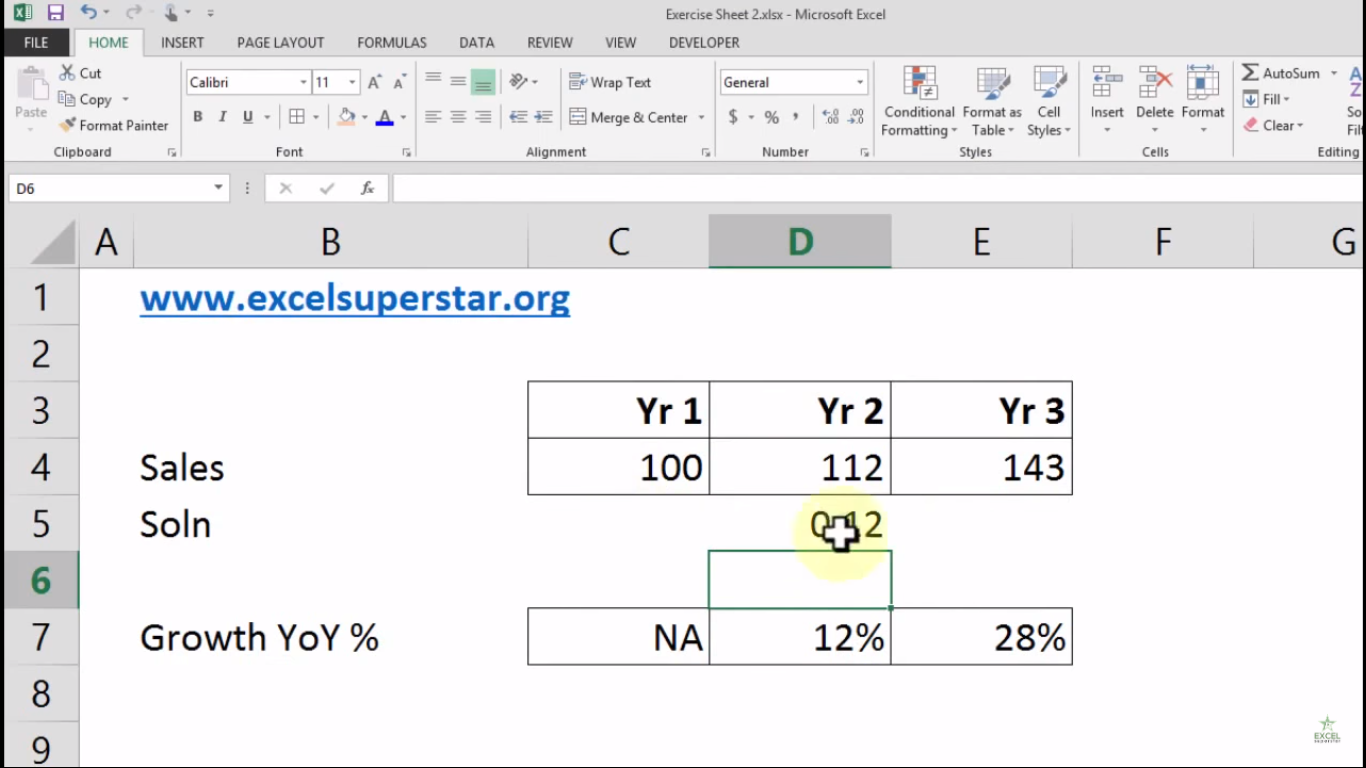5. Now, go to Home Tab and click on the Percentage symbol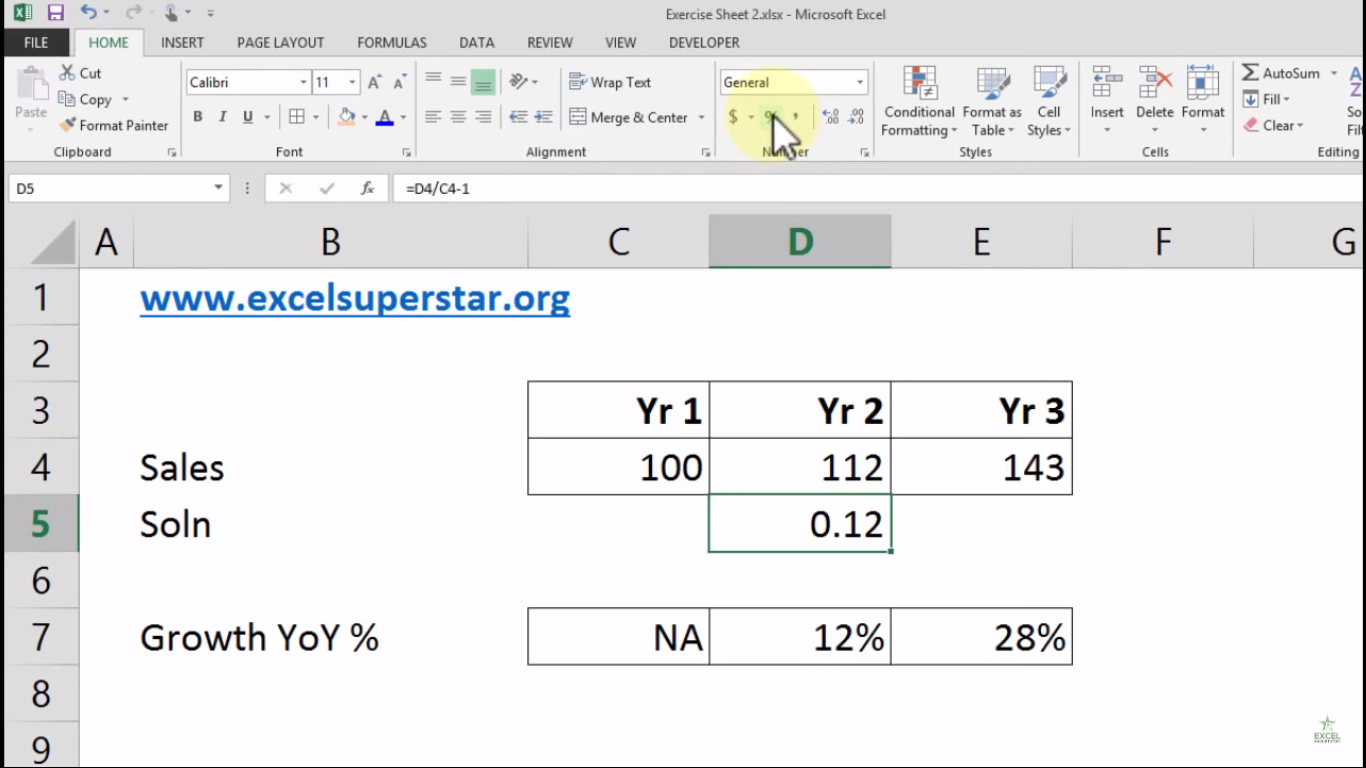6. The answer will be converted into percentage which is 12%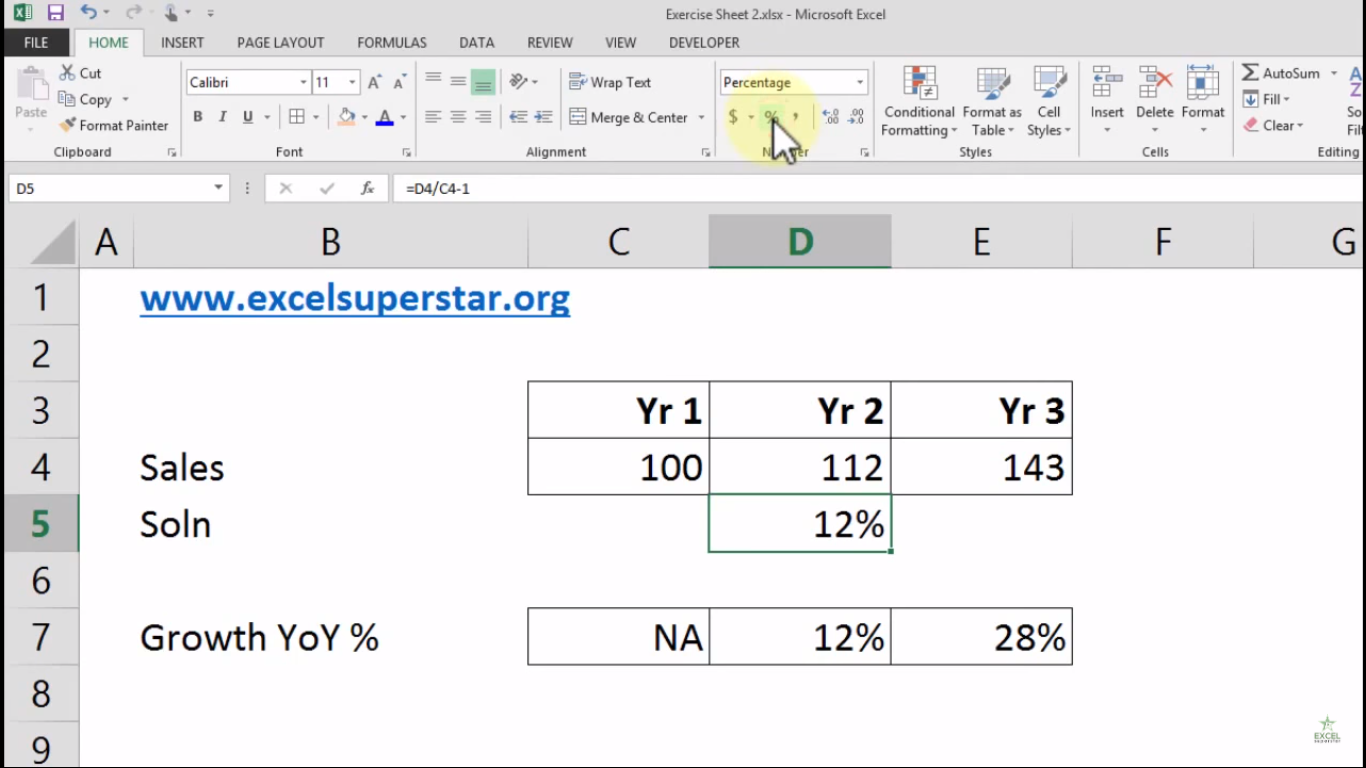7. Drag the cell to the right side to get the answer of next Year on Year Growth Percentage